Begin Content Area -->

## Helical, Shmelical...who cares?

Salt Lake City...June 13, 1994

Last Edited on Wednesday, January 14, 1998

I know, I know...just tell me what to buy!!

In order to help you understand the significance of the helical angle in endodontic files I have to start at the beginning and review two significant portions of high school science with you.

Most of what I was taught I too had forgotten and had to get out the books but if you'll bear with me I'll feed it to you in small bites to make it more digestible.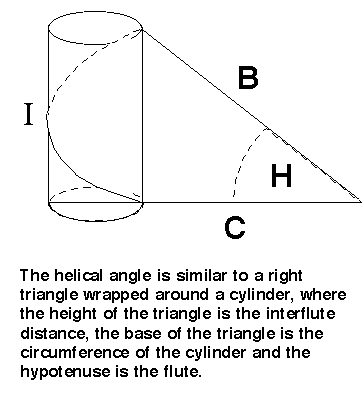Trigonometric functions are ratios between two sides of a right triangle.

A right triangle is on in which one of the sides is a 90 degree or right angle.

As you will recall, the total of all the angles of a triangle must equal 180 degrees, therefore if one of them is 90 the sum of the other two must equal 90.

If you think about it, if there are three sides of a triangle then there are six possible ratios between the lengths of the sides.

If the sides are named A, B, and C then you could have

• I/B

• I/C

• B/I

• B/C

• C/I

• and C/B

In trig these sides of the triangle are not named I,B and C but Opposite, Adjacent and Hypotenuse to reflect their location relative to the angle we refer to.

Each of these ratios has a name, they are

• Tangent = Opposite / Adjacent

• Co-Tangent = Adjacent / Opposite

• Sine = Opposite / Hypotenuse

• Cosine = Adjacent / Hypotenuse

• Secant = Hypotenuse / Adjacent

• Cosecant = Hypotenuse / Opposite

You can visualize the triangle as sitting on one of its non-hypotenuse sides with the right angle to your left, and the angle we are concerned with at the lower right.

The trigonometric tangent is the ratio between the side opposite and the side adjacent, that is between the vertical leg and the side it is sitting on.

All the other ratios you can forget for the moment, because the tangent is the only one we are concerned with here.

If you think about this triangle as a hill on which skiers are enjoying their sport it will help understand some of the endodontic functions better.

We know from high school physics(Newtons Laws of Motion) that every action has an equal and opposite reaction.

And that a body at rest tends to remain at rest(Inertia) unless an external force is applied to it.

We know that gravity acts on the skier pushing downward on the skis and yet the skier somehow goes forward as well as down the hill.

We also know that if there no slope to the hill the skier would go nowhere ( unless he pushes ).

So somehow the hill influences the forward movement.

We also know that the steeper the hill, the faster as skier accelerates.

In fact what causes the skier to move forward is the horizontal force vector of the vertical force of gravity acting against the skis.

If we were able to put a scale between the bindings and the skis we would find out that the skier might weigh 150 pounds on level ground but as soon as he begins accelerating, his weight drops in proportion to the acceleration.

In other words gravity can only exert 150 pounds pressure on him.

And part of that is pushing forward.

The question is...How much?

Your gut feeling might tell us that if we were to double the angle of the hill, the accelerative force would more than double and you would be right.

In fact the horizontal component of force is NOT proportional to the angle of the hill, it is proportional to the TANGENT of the angle of the hill.

The tangent is the ratio between the height of the hill and the length of the base of the hill.

For example, the tangent of a 30 degree angle is 1 divided by 2 because in a 30-60-90 triangle the side opposite the 30 degree angle is half the length of the side adjacent.

If you increase the angle 15 degrees you now have a 45-45-90 triangle and the side opposite equals the side adjacent and the ratio (tangent) is now 1 divided by 1 or 1.0.

Increasing it again by 15 degrees makes the angle 60 degrees, the side opposite double the side adjacent and the tangent is now 2.0.

The point is that as we increase the angle the tangent and thus the amount of horizontal force exerted by gravity increases way out of proportion to the angle.

Now let's return to another high school subject, physics ( actually mechanics ).

Remember the definition of a screw?

A screw is nothing other than an inclined plane wrapped around a cylinder...but of course you knew that, didn't you.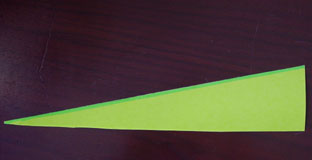If we then take that incline plane(i.e. a right triangle) and wrap it around a cylinder we get a screw.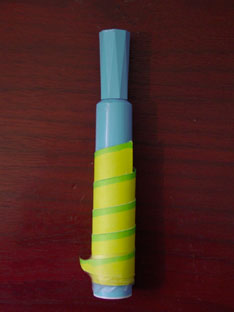But that does not change the nature of the triangle in that it still has the same angles etc.

It is just now a cylinder in three dimensions instead of a plane with two.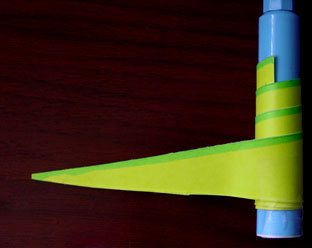So if we take an inclined plane...

or a triangle and wrap it around a cylinder whose circumference is the same length as the base of a triangle we have one helical spiral of a screw.

When you apply a force downward on the same inclined plane it is now referred to as an AXIAL force because it is directed along the long axis of the cylinder.

The amount of force and its horizontal component do not change just because you wrap the inclined plane around a cylinder.

What does change is the direction of the resultant movement.

Instead of moving in a horizontal direction it causes the cylinder to rotate or if you prevent it from rotating it produces torsion.

In the Automated Endo course I demonstrate this concept with a childrens toy...a top the rotates when you push a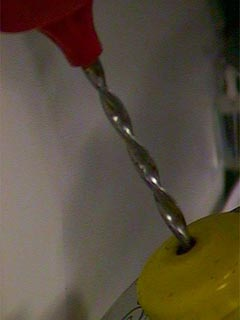helical spiral into it.rotational movement.

An endodontic file is a screw.

When you insert it into a patient's tooth, engage dentin with it's blades and pull on it you are producing an axial load which creates torque and attempts to cause movement.

Since the patient's head will not spin and you are holding onto the file, the torque produces tortional distortion in the file itself.

Endo files are made of wire.

Wire has a lot of tensile strength after all they hang suspension bridges on wire.

But it has very little torsional strength.

So if you twist a wire it will break fairly easily.

So even if you don't twist a file, it distorts in a torsional way due to the resultant torsional deformation due to the angle of the blade.

And the greater the angle the greater the torsion.

It is this torsion that breaks files and that happens when the torsional force exceeds the torsional strength of the metal.

The ideal helical angle is zero degrees because none of the axial load applied to a file results in torsion.

As soon as the angle of the blade exceeds zero there is torsion.

When the angle is 30 degrees the proportion of the axial load that is translated into torque is about 30 per cent.

When the angle reaches 45 degrees it is 50 percent and when the angle reaches 60 degrees it is about 80 percent. Eighty percent of the force that you use to dislodge a stuck file is translated into torque.

So it behooves us to keep the angle low.

How can that be done?

The problem is that endo files are not cylinders as in the example above.

They are cones.

The defining characteristic of a cone is that the diameter and thus it's circumference decreases as you go from bottom to top.

In the case of an endo file, from shank to tip.

Using our example of the screw above, the assumption is made that each flute of the file is created by the hypotenuse of the triangle, the interflute distance is the height of the triangle and the circumference is the length of the base.

So as we go from shank to tip on a file, if the inter-flute distance remains the same i.e. the height of the triangle, and the circumference of the file decreases i.e. the base of the triangle, the angle increases as you proceed from shank to tip.

When the angle increases so does the tangent of the angle, so does the proportion of stress passed to the file.

Since the tip is the weakest point, the thinnest point, the part that has the greatest amount of torque and is the part that get stuck is it any wonder that files break?

In order to minimize the torque at the tip of a file we have to keep the ratio between the height of the incline plane and the base of the incline plane the same.

In this way the ratio is the same.

And the helical angle is the same at the tip as at the shank.

The only file being made that has this feature is the Fine-Cut files from Special Products.

If you pick up a Fine-Cut File you will notice that it looks very much like a Hedstrom file.

It IS similar in one respect.

It has a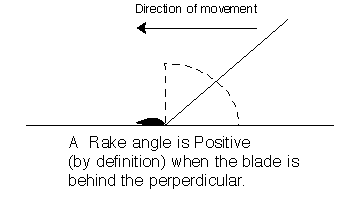positive rake angle on the blades.

That is, it cuts on the way out and does not cut on the way in.

But there the similarity stops.

As you proceed from shank to tip on a Fine-Cut file you will notice that the flutes get closer and closer together.

This has the effect of keeping the ratio between the inter-flute distance and the circumference about the same and thus there is no greater torque at the tip of a size 15 than at the shank of a size 50.

Since we start out with a very low 23 degree helical angle only about 28 per cent of the axial load gets translated into torque and the positive rake angle chisels away any dentin before the metal gives way.

One of the side benefits of a diminishing interflute distance is that the flutes also get shallower as the file gets smaller, thus keeping more strength in the file at the tip.

This gives the user a tremendous advantage over conventional Hedstrom files in that contrary to the warning about Hedstrom files, i.e. never get them stuck, we encourage it.

In fact there is a specific technique for probing what we call dentin pipes that involves repeatedly getting a file stuck in the canal and then pulling outward on it with considerable force. (see "Probing Two Concepts in Course Index")